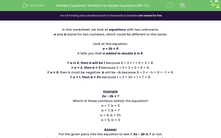# Equations: Solutions to Harder Equations with Two Unknowns

In this worksheet, students must find pairs of numbers that satisfy a harder equation with two unknowns.Key stage:  KS 2

Curriculum topic:   Algebra

Curriculum subtopic:   Use Equations

Popular topics:   Algebra worksheets

Difficulty level:#### Worksheet Overview

In this worksheet, we look at equations with two unknowns.

a and b stand for two numbers, which could be different or the same.

Look at this equation.

a + 2b = 8

It tells you that a added to double b is 8.

If a is 6, then b will be 1 because 6 + 2 × 1 = 6 + 2 = 8.

If a = 2, then b = 3 because 2 + 2 × 3 = 2 + 6 = 8.

If a = 1, then b = 3½ because 1 + 2 × 3½ = 1 + 7 = 8.

Example

3a - 2b = 7

Which of these solutions satisfy the equation?

a = 7, b = 0

a = 7, b = 7

a = 4, b = 2½

a = 5, b = 3

Put the given pairs into the equation to see if 3a - 2b is 7 or not.

In each case, we work out 3 × a take away 2 × b to see if the answer is 7 or not.

 a = 7, b = 0 does not fit because 3 × 7 - 2 × 0 = 21 - 0 = 21 (not 7) a = 7, b = 7 fits because 3 × 7 - 2 × 7 = 21 - 14 = 7 a = 4, b = 2½ fits because 3 × 4 - 2 × 2½ = 12 - 5 = 7 a = 5, b = 3 does not fit because 3 × 5 - 2 × 3 = 15 - 6 = 9 (not 7)

### What is EdPlace?

We're your National Curriculum aligned online education content provider helping each child succeed in English, maths and science from year 1 to GCSE. With an EdPlace account you’ll be able to track and measure progress, helping each child achieve their best. We build confidence and attainment by personalising each child’s learning at a level that suits them.

Get started